# Differentiation rule for piecewise definition by interval

(diff) ← Older revision | Latest revision (diff) | Newer revision → (diff)
Jump to: navigation, search

## Statement

### Everywhere version

Suppose$f_1$ and$f_2$ are functions of one variable, such that both of the functions are defined and differentiable everywhere. Consider a function$f$, defined as follows: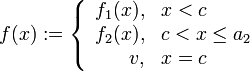$f(x) := \left\lbrace \begin{array}{rl} f_1(x), & x < c \\ f_2(x), & c < x \le a_2 \\v, & x = c \end{array}\right.$

Then, we have the following for continuity:

• The left hand limit of$f$ at$c$ equals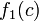$f_1(c)$.
• The right hand limit of$f$ at$c$ equals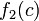$f_2(c)$.
•$f$ is left continuous at$c$ iff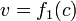$v = f_1(c)$.
•$f$ is right continuous at$c$ iff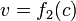$v = f_2(c)$.
•$f$ is continuous at$c$ iff$f_1(c) = f_2(c) = v$.

We have the following for differentiability:

•$f$ is left differentiable at$c$ iff$v = f_1(c)$, and in this case, the left hand derivative equals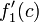$f_1'(c)$.
•$f$ is right differentiable at$c$ iff$v = f_2(c)$, and in this case, the right hand derivative equals$f_2'(c)$.
•$f$ is differentiable at$c$ iff (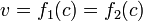$v = f_1(c) = f_2(c)$ and$f_1'(c) = f_2'(c)$), and in this case, the derivative equals the equal values$f_1'(c)$ and$f_2'(c)$.

### Piecewise definition of derivative

If the conditions for differentiability at$c$ are violated, we get the following piecewise definition for$f'$, which excludes the point$c$ from its domain:$f'(x) := \left \lbrace \begin{array}{rl} f_1'(x), & x < c \\ f_2'(x), & x > c \\\end{array}\right.$

If the conditions for differentiability at$c$ are satisfied, we get the following piecewise definition for$f'$, which includes the point$c$ in its domain:$f'(x) := \left \lbrace \begin{array}{rl} f_1'(x), & x < c \\ f_2'(x), & x > c \\ u, & x = c\end{array}\right.$

where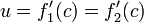$u = f_1'(c) = f_2'(c)$. In particular, the value at$c$ can be included in either the left side or the right side definition.

### Version for higher derivatives

Suppose$f_1$ and$f_2$ are functions of one variable, such that both of the functions are defined and$k$ times differentiable everywhere (and hence in particular the functions and their first$k-1$ derivatives are continuous), for some positive integer$k$. Consider the function:$f(x) := \left\lbrace \begin{array}{rl} f_1(x), & x < c \\ f_2(x), & c < x \le a_2 \\v, & x = c \end{array}\right.$

Then,$f$ is$k$ times differentiable at$c$ if we have all these conditions:$f_1(c) = f_2(c) = v$,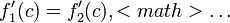$f_1'(c) = f_2'(c), $\dots$,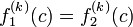$f_1^{(k)}(c) = f_2^{(k)}(c)$. In other words, the values should match, and the values of each of the derivatives up to the$k^{th}$ derivative should match. In that case, the$k^{th}$ derivative of$f$ at math>c$ equals the equal values$f_1^{(k)}(c) = f_2^{(k)}(c)$.

The general piecewise definition of$f^{(k)}$ is, in this case: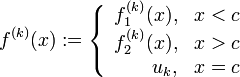$f^{(k)}(x) := \left \lbrace \begin{array}{rl} f_1^{(k)}(x), & x < c \\ f_2^{(k)}(x), & x > c \\ u_k, & x = c\end{array}\right.$

where$u_k = f_1^{(k)}(c) = f_2^{(k)}(c)$.

### Local generalization

The above holds with the following modification: we only require$f$ to be defined as$f_1$ on the immediate left of$c$ (i.e., on some interval of the form$(c - \delta,c)$ for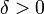$\delta > 0$ and as$f_2$ on the immediate right of$c$ (i.e., on some interval of the form$(c,c + \delta)$ for$\delta > 0$). Further, we only require that$f_1$ and$f_2$ be defined and differentiable on open intervals containing$c$, not necessarily on all of$\R$.